# A Riemann Solver For the Euler Equations of Gas Dynamics

## by John Weatherwax

Introduction
Here you'll find code in FORTRAN implementing a Riemann solver for the Euler equations of gas dynamics. The Euler equations of gas dynamics are given by the following system of three partial differential equations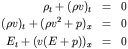Where E is the total energy, i.e. the sum of the kinetic plus the potential (internal) energy given by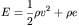For a gamma gas law (ideal gas) it can be shown that the specific internal energy e is given by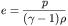where gamma is a given constant (for air it is about 1.4). With all of these definitions the system above is closed in terms of the three conservative variables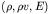or the three primitive variables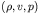A Riemann problem is the solution to the above partial differential equations with a discontinuous initial condition given by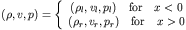The Riemann solver itself is contained in the file "riemann_eu.f", but a simple driver application is compiled when one builds the package. The driver application is called "ne.out" and reads its input from a text file called "newt.inp". In this text file one specifies the left and right states, a Courant like number for use in printing, and some flags that control output. Currently the code is set to solve the "Sod" Riemann problem with initial conditions given by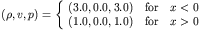The current flags settable in newt.inp are (they can be turned on or off)
• PRINT_NEWTON: Prints to the screen the initial state and the pressure updates computed as the newton iterations converge (at each timestep) as the intersection of the two wave curves is found in the pressure velocity plane. Note: the intersection of the wave curves is found by considering pressure to be an independent variable and velocity the dependent variable.
• PRINT_NEWTON_CONV: Prints the convergence steps of the newton iterations into two files "pu_conv_l_test.dat", and "pu_conv_r_test.dat" representing the newton iterates on each of the two wave curves (left and right) in the pressure velocity space respectively.
• PRINT_RP: Prints a summary of all the wave produced at for the specific Riemann problem to be solved. This is saved in the file "RP_test.dat". The information includes the states between the three waves, the wave speeds, the wave types (shock, rarefaction fan, or contact) and a flag variable denoting the "significance" of the waves.
• PRINT_WC: Prints the left and right wave curves. Specifically their projections onto the (pressure,velocity) plane (in the files pu_curv_{l,r}_test.dat), the (pressure,density) axis (in the files pr_curv_{l,r}_test.dat), and the left and right Riemann invariants (in the file iLiR_curv_{l,r}_test.dat). The ordering of the variables in the files is given by the ordering in the filename. We define the left and right Riemann invariants below.
• PRINT_INIT_WC: Currently not used.
Note an input to the routine riemann_eu.f requires the sound speed of the state to each side of the interface. This is given by (and can be computed with)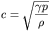As an output from this Riemann solver we also produce the left and right Riemann invariants for this system. The left going Riemann invariant is constant on paths governed by the left facing characteristics (ones traveling on dx/dt=u-c) and is also called a 3-Riemann invariant since it is constant across 3-integral waves. For the Euler equations they are defined by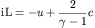The right Riemann invariant is constant on paths governed by the right going characteristics (dx/dt=u+c) and is also called a 1-Riemann invariant since it is constant across 1-integral waves. For the Euler equations they are defined by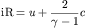Note that these definitions of the Riemann invariants are twice that found in the book:
Supersonic Flow and Shock Waves
by R. Courant and K. O. Friedrichs
Interscience Publishers, Inc. New York, 1948
For further information one can also see the book:
Finite Volume Methods For Hyperbolic Problems
by Randall J. LeVeque
Solving the above Riemann problem produces the following left and right wave curves (the wave curve through the left state is the red curve and the wave curve through the right state is the blue curve)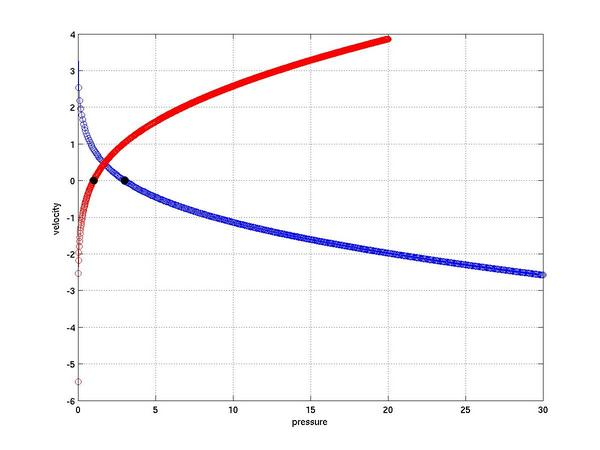With plots of the waves released in velocity at one unit of time from the initial conditions (the central node tracks the location of the contact) look like: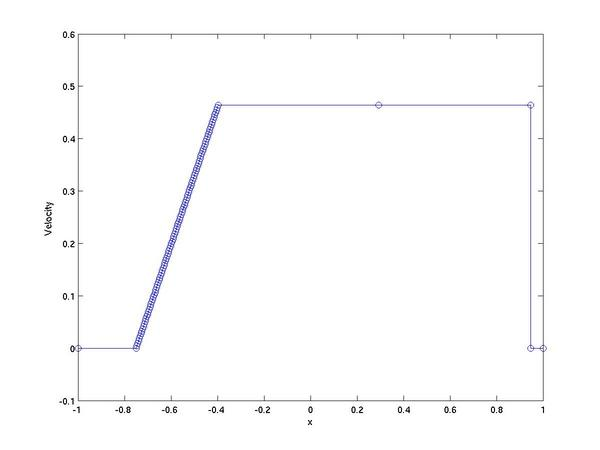The plot of pressure at one unit of time than the initial conditions (the central node tracks the location of the contact) look like: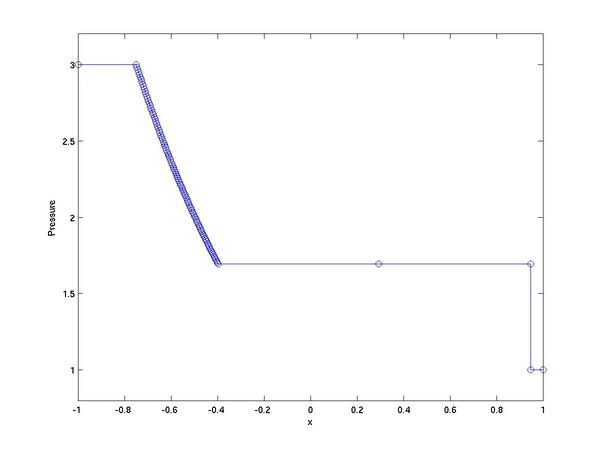The plots in density at one unit of time from the initial conditions look like: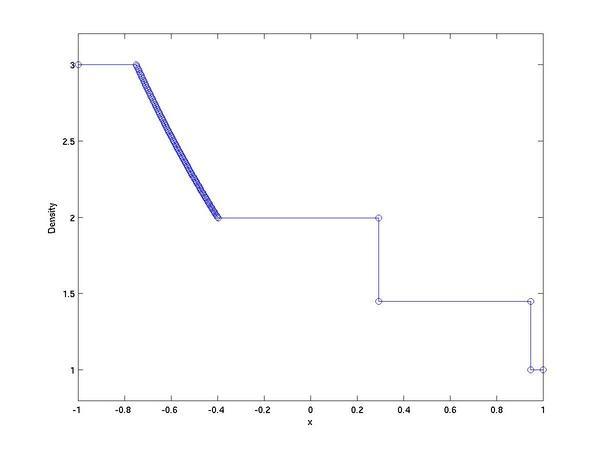When the delivered code is built it contains an additional program to compute the wave curves centered at a given state. The program is called "twc.out" Finally, here is the code
• Euler Riemann solver version 1.0 (.tar.gz) (.zip)
• A simple Matlab function to extract information from the wave curve files is given here
• A simple Matlab function to plot the output of the Riemann solver is given here
As always, I am interested in hearing back if any errors are found to exist.
John Weatherwax